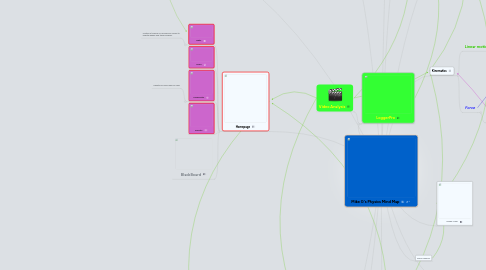# Mike G's Physics Mind Map

Get Started. It's FreeMike G's Physics Mind Map## 1. Right triangle trig

### 1.2. R=Vt^2*sin2(theta)/g

1.2.1. Vt=Sqrt(R*G/sin2(theta)

## 2. Kinematics

### 2.1. Linear motion

2.1.1. Motion Graphs

2.1.1.1. Position

2.1.1.2. Velocity

2.1.1.3. Acceleration

2.1.2. Motion Diagram

2.1.3. Pf = Pi + V*dt

2.1.3.1. r2= r1 + V1(t2-t1) + 1/2a(t2-t1)^2

2.1.3.2. V2= V1 + a(t2-t1)

### 2.2. Force

2.2.1. Newton's 3 Laws

2.2.1.1. I. Every object in a state of uniform motion tends to remain in that state of motion unless an external force is applied to it.

2.2.1.2. II. The relationship between an object's mass m, its acceleration a, and the applied force F is F = ma. Acceleration and force are vectors (as indicated by their symbols being displayed in slant bold font); in this law the direction of the force vector is the same as the direction of the acceleration vector.

2.2.1.3. III. For every action there is an equal and opposite reaction.

3.1.1. Balloon

## 4. VPython

### 4.1. Lesson1/2

4.1.1. Lessons 3,4,5

## 5. Homepage

### 5.1. Dipity

5.1.1. created a timeline of my physics course to update weekly and track progress

### 5.3. MindMeister

5.3.1. Update My mind map for class

## 14. Project 3

### 14.1. Rotational Inertia(moment of intertia)

14.1.1. I(end)=mL^2/3[shaft]

### 14.2. Magnus Effect

14.2.1. Golf ball dimples

### 14.4. Energy

14.4.1. E(KR)=1/2IW^2

### 14.6. Momentum

14.6.1. P=mv-----> deltaP=m*deltav

14.6.1.1. Rolling with a punch reduces force by slowing down the punch